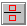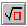# Natural Mathematical Notation

Until now, traditional typesetting and symbolic computation systems forced you to use an array of commands and a complex syntax to represent your input. Many of these systems have over 2,000 separate operators, such as int and diff, that you must learn in order to create input. For example, if you want to integrate the expression
${\int }\frac{{{x}}^{{2}}}{\sqrt{{{x}}^{{2}}-9}}{d}{x}$
using a traditional computation system, you must enter it in linear fashion, int(x\2/sqrt(x\2-9),dx). To typeset it with LATEX you must write $\int\frac{x\{2}}{\sqrt{x\{2}-9}}dx$. A simple typing mistake would cause an error message.

Scientific WorkPlace®, Scientific Word®, and Scientific Notebook® eliminate the need to learn complex syntax by using natural notation for input and to show results. With these products, you can enter mathematics easily with the mouse, or, as you gain confidence and familiarity, with keyboard shortcuts.

Here is how you enter the above integral using the mouse in Scientific WorkPlace, Scientific Word, and Scientific Notebook:

 Entering an integral using the mouse Step # Action Result 1. Click${\int }$ 2. Click3. Type x, click, type 2 4. Click in the denominator box, click5. Repeat step #3, click to the right ${\int }\frac{{{x}}^{{2}}}{\sqrt{{{x}}^{{2}}}}$ 6. Press space, type -9, click to the far right ${\int }\frac{{{x}}^{{2}}}{\sqrt{{{x}}^{{2}}-9}}$ 7. Type dx ${\int }\frac{{{x}}^{{2}}}{\sqrt{{{x}}^{{2}}-9}}{d}{x}$

You can also enter the integral using only the keyboard. Here we use "Ctrl+i" to mean hold down the Ctrl key while you press the "i" key:

 Entering an integral using the keyboard Step # Type Result 1 Ctrl+i (on Windows) Cmd+i (on Macintosh) ${\int }$ 2 Ctrl+f 3 x, Ctrl+up arrow, 2 4 space Moves the insertion point out of the exponent 5 Tab Moves the insertion point to the denominator 6 Ctrl+r 7 Repeat steps 3 and 4 ${\int }\frac{{{x}}^{{2}}}{\sqrt{{{x}}^{{2}}}}$ 8 space, -9 ${\int }\frac{{{x}}^{{2}}}{\sqrt{{{x}}^{{2}}-9}}$ 9 space space Moves the insertion point out of the radical, then the fraction 10 dx ${\int }\frac{{{x}}^{{2}}}{\sqrt{{{x}}^{{2}}-9}}{d}{x}$

In Scientific WorkPlace, Scientific Word, and Scientific Notebook, the space key always moves the insertion point out of the object it is in, and the Tab key always moves the insertion point to the next input box in the current template, if there is one. Thus, in step 9, the first space moves the insertion point out of the radical, but leaves it in the denominator of the fraction. The second space moves it out of the fraction. Pressing the Ctrl key together with the up or down arrow key moves the insertion point up or down to a superscript or a subscript position. The space key returns the insertion point to the main line. Ctrl+up arrow followed by Ctrl+down arrow moves the insertion point to the subscript of a superscript position, not to the main line.

All the symbols in the main TEX fonts are available in Scientific WorkPlace, Scientific Word, and Scientific Notebook, which means you have everything you need to type mathematics. Also, if you know the TEX names for mathematical objects and symbols, you can use them (for example, holding down Ctrl while you type int enters an integral). You do not need to know TEX names to enter mathematics.

MacKichan Software, Inc. 19689 7th Avenue NE, Suite 183 #238 Poulsbo, WA 98370
Phone: 360-394-6033 Toll-free: 877-724-9673 Fax: 360-394-6039